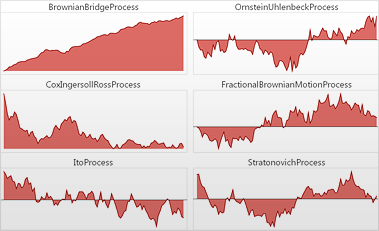# Random Processes

Mathematica 9 adds broad support for random processes, which model systems that evolve over time in a random rather than deterministic fashion, but where states that are near in time are dependent. Random processes, like distributions, are symbolic expressions and can be simulated or estimated from data, and the value of a process at any point in time is a distribution and can be fully used as a distribution. Mathematica 9 fully supports a large number of random processes with some larger groups including parametric processes, finite Markov processes, queueing processes, time series processes and stochastic differential equation processes.• Directly simulate one or several paths for any process.
• Easily work with regular or irregular, scalar or vector, single or multiple time series data.
• Automatically estimate process parameters from time series data.
• Work with single or multiple time slices of processes as distributions.
• Directly compute slice statistics such as mean, median, and variance function, etc.
• Directly compute covariance, correlation, and absolute correlation functions.
• Use random processes to compute general probabilities and expectations.
• Full support for symbolic, numeric, and statistical methods for computing with processes.
• Fully support a large number of parametric random processes. »
• Full support for discrete-time and continuous-time finite Markov processes. »
• Full support for general queue and network queue processes. »
• Full support for time series processes. »
• Full support for stochastic differential equation processes. »

## Mathematica

Questions? Comments? Contact a Wolfram expert »